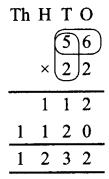# DAV Class 3 Maths Chapter 4 Worksheet 6 Solutions

The DAV Class 3 Maths Book Solutions and DAV Class 3 Maths Chapter 4 Worksheet 6 Solutions of Multiplication offer comprehensive answers to textbook questions.

## DAV Class 3 Maths Ch 4 WS 6 Solutions

Question 1.
24 × 17
Solution:
24 × 17Question 2.
86 × 13
Solution:
86 × 13Question 3.
29 × 40
Solution:
29 × 40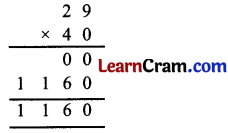Question 4.
19 × 43
Solution:
19 × 43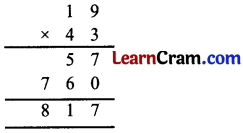Question 5.
35 × 36
Solution:
35 × 36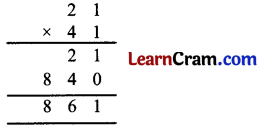Question 6.
28 × 28
Solution:
28 × 28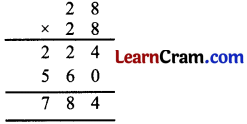Question 7.
61 × 31
Solution:
61 × 31Question 8.
84 × 23
Solution:
84 × 23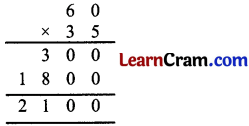Question 9.
50 × 99
Solution:
50 × 99Question 10.
21 × 41
Solution:
21 × 41Question 11.
84 × 23
Solution:
84 × 23Question 12.
62 × 51
Solution:
62 × 51### DAV Class 3 Maths Chapter 4 Worksheet 6 Notes

Multiplication (2-digit numbers by 2-digit numbers)

It is done in three steps.

1. Multiply 56 by 2 ones
56 × 2 = 112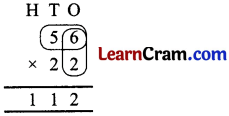2. Next multiply 56 by 2.
3. Then add the two products